American Journal of Applied Mathematics
Volume 4, Issue 2, April 2016, Pages: 92-98

Wavelets in the Analysis of Autoregressive Conditional Heteroskedasticity (ARCH) Models Using Neural Network

Ataulla1, Mohammed Yunus2, Mohammad S. Alsoufi2

1Department of Mathematics, HKBK College of Engineering, Bangalore, India

2Department of Mechanical Engineering, Umm Al-Qura University, College of Engineering and Islamic Architecture, Makkah, Kingdom of Saudi Arabia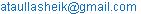(Ataulla)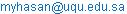(M. Yunus)

Ataulla, Mohammed Yunus, Mohammad S. Alsoufi. Wavelets in the Analysis of Autoregressive Conditional Heteroskedasticity (ARCH) Models Using Neural Network. American Journal of Applied Mathematics. Vol. 4, No. 2, 2016, pp. 92-98. doi: 10.11648/j.ajam.20160402.14

Received: February 29, 2016; Accepted: March 15, 2016; Published: March 30, 2016

Abstract: In the paper, proposed a new method for the time frequency signal analysis, speech processing and other signal processing applications. Stationary signal components can be analyzed by a powerful tool called as Fourier transform. But it is fizzled for analysing the non-stationary signal whereas wavelet transform allows the components of a non-stationary signal to be analyzed. It is the improved version of Fourier transform. Wavelets allow complex information such as music, speech, images and patterns to be decomposed into elementary forms at different positions and scales and subsequently reconstructed with high precision. Here, for extracting the best features of non-stationary signal we use discrete wavelet transform. This can be decomposed into two components named as high frequency component and low frequency component. The decomposed output component is sent for regression analysis. This is done by passing through ARCH model which can characterize and model observed time series. An ARCH time series is the one in which the variance of the error in a period depends on upon size of the squared error in the previous period i.e. if a large error occurs in one period, the variance of the error in the next period will be even larger. The performance of the ARCH will be improved by predicting its co-efficient or cofactor using an artificial technique. The artificial technique presented in this paper is neural network, which is capable of handling sophisticated computations similar to the human brain. The proposed model algorithm will be implemented in MATLAB and the output performances are estimated.

Keywords: Signal Analysis, Discrete Wavelet Transform, Frequency Decomposition, Regression Analysis, ARCH Model, Neural Network

1. Introduction

The power spectral density estimation is explained as, from a finite record of a stationary data sequence, estimate how the total power is distributed over frequencies, or more practically, over narrow spectral bands (frequency bins) . Spectral estimation methods are two types: Classical (Nonparametric) Methods for e.g., Pass the data through a set of band-pass filters and measure the filter output powers and Parametric (Modern) Approaches for e.g., Model the data as a sum of a few damped sinusoids and estimate their parameters. Parametric Methods may offer better estimates if data closely agrees with assumed model. Otherwise, Nonparametric Methods may be better. Several well-known techniques have been developed to carry out signal analysis, most prominently techniques based on Fourier transforms, filter banks, and wavelets .

Wavelet analysis is originally introduced in order to improve seismic signal analysis by switching from Short Time Fourier Analysis (STFT) to new better algorithms to detect and analyze abrupt changes in non linear signals. In comparison to the STFT, wavelet analysis makes it possible to perform a multi-resolution analysis. It can be performed in several ways; a continuous wavelet transform, a discretized continuous wavelet transform and a true discrete wavelet transform . One of the main advantages of wavelet analysis is the ability to perform local analysis  and is capable of decomposing a signal into component wavelets i.e., high frequency component and low frequency component. This Wavelet theory is capable of revealing aspects of data that other signal analysis techniques miss the aspects like trends, breakdown points, and discontinuities in higher derivatives and self-similarity . The fields of wavelet analysis application vary from science, engineering and medicine to finance [6-9].

Autoregressive conditional heteroskedasticity (ARCH) models, introduced by Engle  and generalized by Bollerslev , are widely used in various financial applications such as risk management, option pricing, foreign exchange, and the term structure of interest rates .

An ARCH time series is the one in which the variance of the error in one period depends on size of the squared error in the previous period. The ARCH model provides a rich class of possible parameterization of conditional heteroskedasticity (i.e., time-varying volatility) and explicitly recognizes the difference between the unconditional variance and the conditional variance, allowing the latter to change over time. The fundamental characteristic of this model is that magnitudes of recent innovations provide information about future volatility . Recently, generalized autoregressive conditional heteroskedasticity (GARCH) models have been proposed for modelling speech signals for speech enhancement , speech recognition and voice activity detection applications.

An artificial neural network (NN) is, basically, a non-parametric attempt to model the human brain. NN act like a human brain, trying to recognize regularities and patterns in the data. They can learn from experience and generalize based on their previous knowledge. Neural Networks (NNs) with internal temporal dynamic can be applied to non-linear DSP problems such as speech processing, non-linear filtering and system modeling   and are now the biggest challengers to conventional time series forecasting methods [17-23]. A variety of NNs are available. Generally in NN, we will have input layer, hidden layers and output layer. These layers connected to each other by proper weighted neurons. Before implementing the NN, it needs to go proper training and testing. Here, we will use back propagation for training the NN. After that it is tested to give accurate output (prediction).

In this paper, a new method is presented for non-linear signal analysis. The application of wavelets in the analysis of ARCH model using NN is presented. The proposed method is implementing the discrete wavelet transform for extracting the best features of signal and ARCH model using NN for regression analysis of the signal. Here, related works regarding non-linear signal analysis are described in section 2. The description of the proposed method is explained in Section 3 with analytical expressions. In Section 4, the results and discussion is presented. Finally, in Section 5 the paper is concluded.

2. Related Works: A Brief Review

For power spectral analysis, there were so many research scholars presented so many articles in the literature. The main agenda of power spectral analysis is to estimate the spectral density of a random signal from a sequence of time samples of the signal. It characterizes the frequency content of the signal. The purpose of this estimating is to detect any periodicities in the data, by observing peaks at the frequencies corresponding to these periodicities. It can be performed based upon the known mathematical properties of the signal, or it may be carried out based on time domain measurements of a real signal, using continuous time or discrete time spectral analyzer. Here, we have shown some of the related work done in power spectral estimation as follows.

Petr Sysel et al.  proposed a method of power spectral density estimation using wavelet transform in spectral domain. To reduce periodogram variance the proposed method used the procedure of thresholding the wavelet coefficients of a periodogram. Then the smoothed estimate of power spectral density of noise was obtained using the inverse discrete wavelet transform. It led to a reduced variance in the estimate of power spectral density of noise. It can be said that methods of power spectral density estimation with a smaller variance of estimation (thresholding of wavelet coefficient of peridogram) provide a smaller variance of the improvement of signal-to-noise ratio.

Youngchan Kim et al.  have demonstrated that the wavelet power spectrum estimation technique (WPSET) could be successfully applied to high-resolution THz-TDS. Through the wavelet power spectrum estimation, the fluctuations on high-resolution THz amplitude spectra were removed while maintaining original spectral information. By investigating a THz transmission spectrum of water vapour, the parameters of the spectral singularities were shown to remain unchanged after the processing. They showed that the WPSET was ideally suitable for high-resolution THz-TDS. The WPSET would make high-resolution THz-TDS more practical by enhancing the spectrum quality.

Tariq Abu Hilal et al.  have proposed integrated Discrete Wavelet Transform (DWT) and Logarithmic Power Spectrum Density (PSD) for speaker accurate formants extraction, afterward correlation coefficient was used for feature classification; the correlation thresholding factor was adjusted. As the system works with the recorded samples, the features tracking capability was excellent with text dependant dataset; so the system could be applied in password, PINs identification, security system or mobile phones. They showed an excellent performance, around 95% Recognition Rate.

Wasim Ahmad et al.  have proposed a DWT based shift-invariant transform, which could be used to represent a signal in analysis-synthesis and compression applications, or to extract features in signal detection and classiﬁcation applications. They have used a shift-invariant analysis scheme which was nonredundant. Then this scheme was combined the minimum-phase (MP) reconstruction with the DWT so that the resultant scheme provide a shift-invariant transform. The detailed properties of MP signal and different methods to reconstruct it were explained. The proposed scheme could be used for the analysis-synthesis, classiﬁcation and compression of transient sound signals.

Lakshmanan M.K et al.  have proposed a reconfigurable wavelet packet transceiver for spectral analysis and dynamic spectrum access. The transceiver consists of a Wavelet Packet Spectral Estimator (WPSE) and a Wavelet Packet Multi-carrier Modulator (WPM) both of which were implemented using filter banks. The WPSE estimated the radio environment and identifies spectrum holes and occupied bands. Then this information was used to shape the time-frequency characteristics of the WPM transmission waveform in a way that the occupied bands were evaded. This was done by vacating those sub-carriers which lie in and around the occupied bands. The WPSE used the same filter bank structure as used for WPM data modulation and hence doesn't added to the implementation costs. Through computer simulations the operation of the proposed system was demonstrated. Those performances were also compared with two other candidate systems based on Fast Fourier Transform (FFT) and Orthogonal Frequency Division Multiplexing (OFDM). They showed that WPSE/WPM, in relation to FFT/OFDM, offers better bit-error-rate (BER) performance and bandwidth efficiency. This was facilitated by the excellent time-frequency localization of wavelet filters which resulted in better estimation of spectrum and spectral confinement of transmission waveform.

Fulufhelo V et al.  have presented a computational intelligence approach for predicting missing data in the presence of concept drift using an ensemble of multi-layered feed forward neural networks. An algorithm that detected concept drift by measuring heteroskedasticity was proposed. Six instances prior to the occurrence of missing data were used to approximate the missing values. The algorithm was applied to simulated time series data sets resembling non-stationary data from a sensor. Results showed that the prediction of missing data in non-stationary time series data was possible but was still a challenge. For one test, up to 78% of the data could be predicted within 10% tolerance range of accuracy.

Carmen Vidal et al.  have proposed a hierarchical mixture of autoregressive (AR) models for the analysis of nonlinear time-series. The model was a decision tree with soft sigmoidal splits at the inner nodes and linear autoregressive models at the leaves. The global prediction of the mixture was a weighted average of the partial predictions from each of the AR models. The weights in this average were computed by the application of the hierarchy of soft splits at the inner nodes of the tree on the input, which consists in the vector of the delayed values of the time series. The weights could be interpreted as a priori probabilities that an example was generated by the AR model at that leaf. As an illustration to the flexibility and robustness of the models generated by these mixtures, an application to the analysis of a financial time-series was presented.

Junghwan Jin et al.  employed wavelet decomposition and the ARIMA, GARCH, and ANN models, to forecast the Henry Hub weekly gas spot price. The importance of the boundary problem of wavelet decomposition is illuminated by applying two different decomposition approaches. In addition, the effect of detail components in forecasting is analyzed by comparing the forecasting results with or without the detail components.

3. Proposed Methodology

In the paper, the application of wavelet transform is used for power signal spectral estimation by ARCH model. Here, we use discrete wavelet transform to decompose the signal into two components i.e., high frequency component and low frequency component. Then, it is sent for regression analysis by ARCH model. The performance of ARCH model is improved by Artificial Intelligence technique. The artificial intelligence technique presented in this paper is Neural Network (NN) which is used for predicting the ARCH model coefficients. The computational power of the proposed algorithm is reduced by NN. The elucidation of the proposed algorithm is described in the next section. Before that, the block diagram of proposed method is shown in figure 1.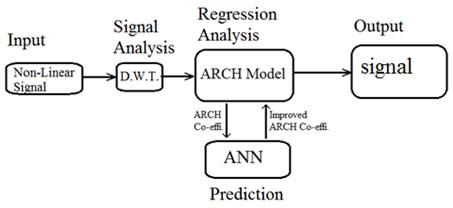Figure 1. The block diagram of proposed method.

3.1. Feature Extraction of Non-Linear Signal by Wavelet Transforms

The transform of a signal is just another form of representing the signal. It does not change the information content present in the signal. Time-frequency representation of the signal can be represented by the Wavelet Transform. It was developed to reduce the problems involved with the Short Time Fourier Transform (STFT), which can also be used to analyze non-stationary signals. While STFT gives a constant resolution at all frequencies, the Wavelet Transform uses multi-resolution technique by which different frequencies are analyzed with different resolutions. There are two types of wavelet transforms; they are continuous wavelet transform (CWT) and discrete wavelet transforms (DWT). In CWT, the signals are analyzed using a set of basic functions which relate to each other by simple scaling and translation. In the case of DWT, by using digital filtering techniques a time-scale representation of the digital signal is obtained. The signal to be analyzed is passed through filters with different cut-off frequencies at different scales. The Wavelet Transform, at high frequencies, gives good time resolution and poor frequency resolution, while at low frequencies; the Wavelet Transform gives good frequency resolution and poor time resolution.

In the proposed method, discrete wavelet transform is used for decomposition of frequency component of the signal. Here, for analyzing the signal it uses the wavelet function and scaling function. Now, the given signal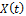is decomposed on multi-resolution levels as follows: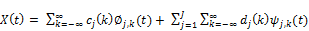(1)

Where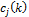denotes the approximated signals or scaling coefficients,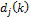represents the detailed signals or wavelet coefficients at each level of decomposition and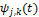is the wavelet function andis the scaling function.

They are defined as: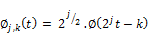(2)(3)

DWT has the capability of decomposing a signal into an approximation and detail information. Thus show that it can analyze the signal at different frequency ranges with different resolutions. The digital filters to be used for decomposition are low pass filter and high pass filter. Here,andare calculated by digital filtering operations and scaling function associates with low pass filter where as wavelet function associates with high pass filter. This allows the decomposition of the signal with different frequency bands by recursively applying filters to the signal. Then the signal is decomposed into its high and low frequency components referred as details and approximations respectively. Then, the decomposed frequency components are sent to regression analysis. This is done by ARCH model and explained in the next section.

3.2. Regression Analysis by ARCH Model

The decomposed component of the discrete wavelet transform is given as input to the ARCH model for regression analysis. Here, the given input information is discrete wavelet functionavailable at timeand we have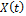as real-valued discrete-time stochastic process. The output of the ARCH model after regression analysis can be written as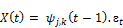(4)

Where,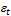is a white-noise disturbance term with variance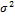. Then, the innovation (prediction error)at timein the minimum mean-squared error sense is obtained by subtracting fromits conditional expectation given the information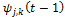,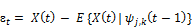(5)

The conditional variance (volatility) ofgivenis by definition the conditional expectation of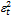,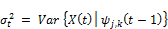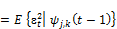(6)

As we can see, the conditional variance depends on various ‘n’ time values of, and then the process is an ARCH (n) process, and can be written as: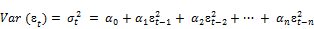(7)

Where,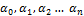are the coefficients of the ARCH model.

ARCH model coefficients play a key role in the regression analysis of signal. The complexity of this process is reduced by using a neural network. The participation of Neural Network in the proposed method is described in the following section.

3.3. Performance Estimation of Proposed Method Is Increased by NN

The neural network plays an important role in signal processing for improving the performance of ARCH model by predicting its coefficients effectively for better spectrum estimation. Here, the purpose of the artificial intelligence technique is to predict the improved ARCH model coefficients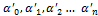from given ARCH model coefficients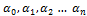. In the proposed paper, the feed forward type neural network is used; it consists of three layers that are named as input layer, hidden layer and output layer. Here, the input of the neural network is denoted as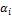(where i = 1, 2…n), the output is denoted as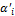(where i = 1, 2…n). Structure of Neural Network of the NN is shown in figure 2.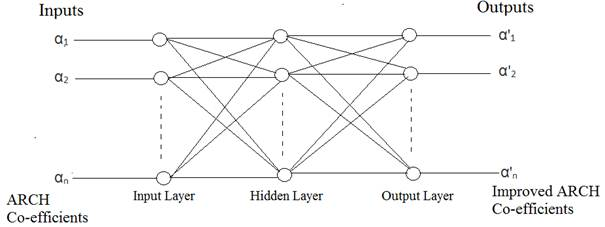Figure 2. Structure of neural network.

The proposed structure of the neural network is described as follow. In the figure 2, the input layer, hidden layer and output layer of the network are U11, U12 U1n, U21, U22…U2n, U31, U32…U3n respectively. The weight of the input layer to hidden layer is denoted as V11, V12…V1n, V21, V22, V2n, Vn1, Vn2, and Vnn respectively. The weight of the hidden layer to output layer is denoted as V211, V212, V21n, V221, V222, V22n, V2n1, V2n2 and V2nn respectively.

The Back Propagation (BP) training algorithm is used to train NN. The training process of NN is described as follows; Initialize the input, output and weight of each neuron. Here, αi is the input of the network and α’i is the output of the network. The inputs of training dataset areto the classifier and determine the Back Propagation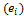error as follows,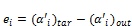(8)

In above equation (8),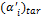is target output and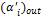is the network output. Then, the network output is calculated as follows: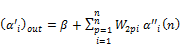(9)

Where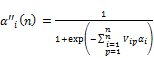(10)

Above two equations (9) and (10) show the active functions of output layer and hidden layer respectively. Then adjust the weight of all neurons as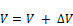(11)

Where,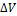is the change of weight which can be determined as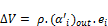(12)

In above equation (12),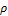is the learning rate, usually it is 1. Repeat the above process, until Back Propagation error () gets minimized to a least value i.e., 10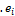<1. Once the process gets completed, the network is well-trained and it would be suitable for providingvalues for any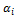. Then, the performance of the proposed method is estimated with ARCH model using Neural Network.

4. Results and Discussion

The application of wavelets in the analysis of ARCH model using NN was implemented in MATLAB working plat and the version is 7.12 for analyzing the non-linear signal i.e., to estimate the power spectral density of a randomised signal from a sequence of time samples of the signal. First of all, we used Discrete Wavelet Transform for extracting the best features of non-linear signal. The system input signal (its amplitude according to time samples) was given to DWT and is shown in figure 3.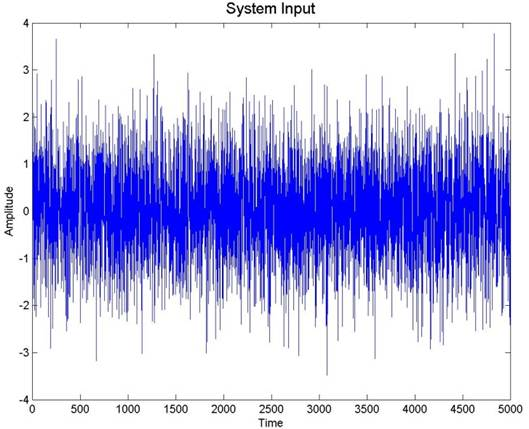Figure 3. Input to the DWT.

DWT was capable of decomposing the system input signal into two components called as high frequency component and low frequency component which could be referred as details and approximations of the signal information. Decomposition of the signal was done by using digital filters such as high pass filter and low pass filter for high and low frequency component respectively and was showed in figure 4 and 5.

Then, the decomposed frequency components were sent to regression analysis. This was done by ARCH model. This model used the decomposed frequency component i.e., wavelet function for regression analysis. The output of ARCH model after regression analysis was showed in figure 6.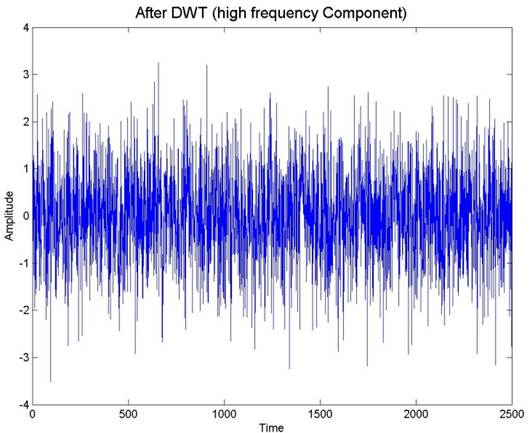Figure 4. High frequency component of signal after decomposition by DWT.Figure 5. Low frequency component of signal after decomposition by DWT.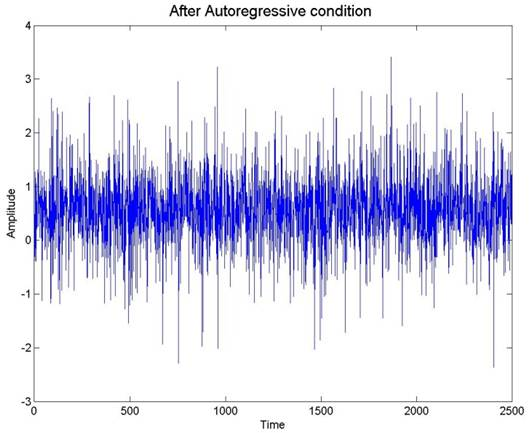Figure 6. ARCH model output.

After regression analysis, the randomized signal was obtained as output. Here, coefficients of the ARCH model played a key role in analysis. For improving the performance of this ARCH model, NN was used which could predict the improved coefficients of ARCH model from its previous coefficients. These improved coefficients perform better than the previous one. The system output without and with NN participation were showed in figure 7 and 8. The system output shown in figure 6 is the required non-linear signal.

Then the proposed method for spectral analysis is examined. The analysis is based on the system input signal samples, then the decomposition of the signal and after that regression. The performance of the system output after regression by ARCH model is also examined. Then the performance of ARCH model alone is compared with the system output after regression by ARCH model using NN. The usage of NN with ARCH model reduced the system computational complexity and showed some improvement in the system output.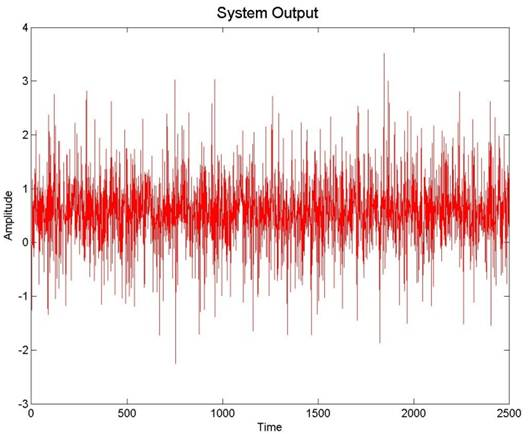Figure 7. System output without neural network.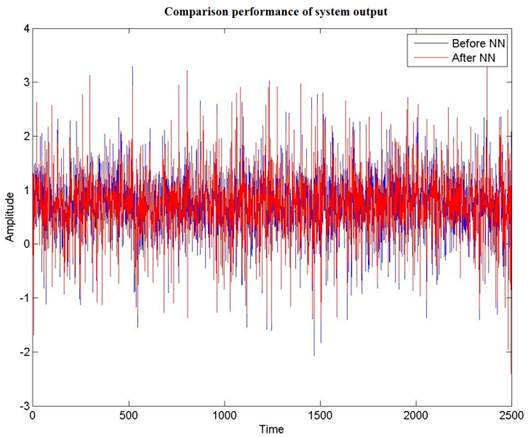Figure 8. System output with neural network.

5. Conclusion

In the paper, proposed method was used for time frequency signal analysis, speech processing and other signal processing applications. Here, the non linear signal to be estimated was first decomposed by DWT into its high frequency and low frequency component. Then it was sent to regression analysis by ARCH model. We used Neural Network for improving the performance of ARCH model by predicting its coefficients. Analytical expressions were showed for wavelet transform, ARCH model and NN. The proposed method was implemented in MATLAB. The performance of the proposed method was illustrated in and showed clearly with simulations. The outputs of the ARCH model alone and ARCH model with NN were showed in and compared. From the comparison of these two outputs, it showed that the power spectral estimation of a random signal was improved.

References

1. Jian Li, "Spectral Estimation", Department of Electrical and Computer Engineering University of Florida Gainesville, FL 32611, USA.
2. Jonas Buchli, Ludovic Righetti and Auke Jan Ijspeert, "Frequency analysis with coupled nonlinear oscillators",Physica D: Nonlinear Phenomena, Volume 237, No. 13, 1 August, Pg 1705–1718, 2008.
3. R.J.E. Merry, "Wavelet Theory and Applications", A literature study, Eindhoven, June 7 2005.
4. M. Misiti, Y. Misiti, G. Oppenheim, and J-M Poggi, "Wavelets Toolbox Users Guide: The MathWorks", Wavelet Toolbox for use with MATLAB, 2000.
5. M. Sifuzzaman, M.R. Islam and M.Z. Ali, "Application of Wavelet Transform and its Advantages Compared to Fourier Transform",Journal of Physical Sciences, Vol. 13, 121-134, 2009.
6. Pascal Yiou, Didier Sornette, Michael Ghil,"Data-adaptive wavelets and multi-scale singular-spectrum analysis", Physica D 142, 254–290, 2000.
7. D.B. Pawar, R.S. Kawitkar, M. Selva Balan, "Wavelet Analysis for Processing of Earthquake Records", International Journal of Science and Technology, Volume 2 No.5, May 2012.
8. G. Ranganathan, R. Rangarajan, V. Bindhu, "ECG Signal Analysis for Mental Stress Assessment using Wavelets and Fuzzy Clustering",European Journal of Scientific Research, Vol.65, No.2, pp. 268-280, 2011.
9. Abdul Karim, Samsul Ariffin, Bakri Abdul Karim, M Tahir Ismail, M Khatim Hasan, Jumat Sulaiman, "Applications of Wavelet Method in Stock Exchange Problem",Journal of Applied Sciences 01/2011.
10. R.F.Engle, "Autoregressive conditional heteroskedasticity with estimates of the variance of united kingdom inflation", Econometrica 50, pp. 987–1007, July 1982.
11. T.Bollerslev, "Generalized autoregressive conditional heteroskedasticity", J.Econometrics 31 (3), 307–327, April 1986.
12. T. Bollerslev, R.Y. ChouKenneth, F. Kroner, ARCH modeling in finance: A review of the theory and empirical evidence, J. Econometrics 52 (1–2), 5–59, April–May 1992.
13. Israel Cohen, "Modelling speech signals in the time–frequency domain using GARCH", Signal Processing 84, 2453–2459, 2004.
14. Israel Cohen, "Speech spectral modeling and enhancement based on autoregressive conditional heteroscedasticity models",Elsevier, Signal processing, Volume 86, Issue 4, Pages 698-709,2006/4/30.
15. P. Campolucci, A. Uncini, F. Piazza, "Fast Adaptive IIR-MLP Neural Networks for Signal Processing Application", Proceedings of IEEE Int. Conference on Acoustic Speech and Signal Processing, Atlanta, USA, 7-10 May 1996.
16. Aurelio Uncini, "Audio signal processing byneural networks", Elsevier / Neurocomputing, 593–625, 2003.
17. RJ Frank, Neil Davey, SP Hunt, "Time series prediction and neural networks", Journal of Intelligent & Robotic Systems, Volume 31, Issue 1, Pages 91-103, 2001/5/1.
18. GP Zhang1and VL Berardi, "Time series forecasting with neural network ensembles: an application for exchange rate prediction", Journal of the Operational Research Society, 52, 652-664, 2001.
19. AK Dhamija, VK Bhalla, "Financial Time Series Forecasting: Comparison of Neural Networks and ARCH Models",International Research Journal of Finance and Economics, Issue 49, 2010.
20. Mohammed Awad, "Chaotic Time series Prediction using Wavelet Neural Network",Journal of Artificial Intelligence: Theory and Application, Vol.1, Iss.3, pp. 73-80, 2010.
21. Juan Peralta, Xiaodong Li, German Gutierrez, Araceli Sanchis, "Time series forecasting by evolving artificial neural networks using genetic algorithms and differential evolution", IEEE World Congress on Computational Intelligence, 18-23, July-2010.
22. A. Lendasse, E. De Bodt, V. Wertz, And M. Verleysen, "Non-linear financial time series forecasting – Application to the Bel 20 stock market index", European Journal of Economic and Social Systems 14 N° 1, 81-91, 2000.
23. Hanh H. NguyenÆChristine W. Chan, "Multiple neural networks for a long term time series forecast", Neural Comput & Applic No.13, 90–98, 2004.
24. Petr Sysel, Jiri Misurec, "Estimation of Power Spectral Density using Wavelet Thresholding", Proceedings of the 7th WSEAS International Conference on CIRCUITS, SYSTEMS, ELECTRONICS, CONTROL and SIGNAL PROCESSING, 2008.
25. Youngchan Kim, Kyung Hwan Jin, Jong Chul Ye, Jaewook Ahn, and Dae-Su Yee, "Wavelet Power Spectrum Estimation for High-resolution Terahertz Time-domain Spectroscopy"Journal of the Optical Society of Korea, Vol. 15, No. 1, pp. 103-108, March 2011.
26. Tariq Abu Hilal , Hasan Abu Hilal, Riyad El Shalabi and Khalid Daqrouq, "Speaker Verification System Using Discrete Wavelet Transform And Formants Extraction Based On The Correlation Coefficient", Proceedings of the International MultiConference of Engineering and Computer Scientists, Vol II, 2011.
27. Wasim Ahmad, Hüseyin Hacıhabibo˘gluy, and Ahmet M. Kondoz, "DISCRETE WAVELET TRANSFORM BASED SHIFT-INVARIANT ANALYSIS SCHEME FOR TRANSIENT SOUND SIGNALS", Proc. of the 13th Int. Conference on Digital Audio Effects, September 6-10, 2010.
28. Lakshmanan M.K,Ariananda D.D, Nikookar, H,"A reconfigurable wavelet packet filter bank transceiver for spectral analysis and dynamic spectrum access", IEEE Symposium onCommunication, Networking & Broadcasting ;Computing & Processing, 564-575, 3-6 May 2011.
29. Fulufhelo V. Nelwamondo, Tshilidzi Marwala, "Handling Missing Data from Heteroskedastic and Nonstationary Data" Advances in Neural Networks – ISNN 2007, Lecture Notes in Computer Science Vol. 4491, pp 1293-1302,2007.
30. Carmen Vidal, Alberto Suárez, "Hierarchical Mixtures of Autoregressive Models for Time-Series Modeling" Artificial Neural Networks and Neural Information Processing,Lecture Notes in Computer Science Vol. 2714, pp 597-604, 2003.
31. Junghwan Jin and Jinsoo Kim Forecasting Natural Gas Prices Using Wavelets, Time Series, and Artificial Neural Networks, Journal plosone, November 5,2015. doi: http:\\DOI: 10.1371/journal.pone.0142064.

 Contents 1. 2. 3. 3.1. 3.2. 3.3. 4. 5.
Article ToolsAbstractPDF(1542K)